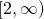# Domains and Rules

When are two functions equal? A function is a kind of relation, which means it's a kind of set. So two functions being equal means, fundamentally, that each is a subset of the other. That's inconvenient, and it doesn't really jibe with our understanding of functions as encoding rules.

Theorem About When Functions Are Equal (TAWFAE). Let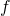and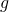be functions. Then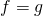if and only if:

1.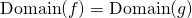, and
2.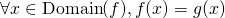.

Proof. This theorem sort of looks like it will be complicated to prove, but let's keep our wits about us and rely on logic to help us out.

Globally, this is a biconditional proof so it has two directions:

(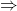) Assume. We need to prove two things:

(clause 1) This is the claim that two sets are equal, so we have to prove each is a subset of the other:

(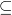) Let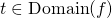. Then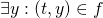. Since, this means. So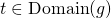.

(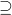) Let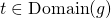. Then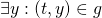. Since, this means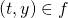. So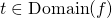.

(clause 2) This is a universal claim, so we start the same way as always: Let. Then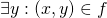. We can rewrite this as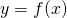. On the other hand, we know from clause 1 that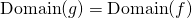, so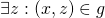. We can rewrite this as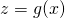. But since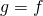, we actually have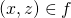.

Since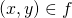and, andis a function,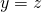.

So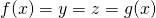.

(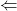) Assume both clauses 1 and 2 hold. In this direction, we need to prove two sets are equal.

() Let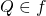. Then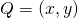for some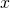and some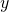.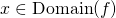, so by clause 1,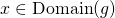. Then there's some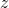with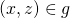. We can rewrite in function notation asand. By clause 2, we know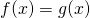, so. That is,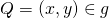.

() This is so similar to, I'll let you work it out.

Since we proved both directions, we're done.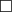.

This theorem gives us a new way to prove two functions are equal, should we ever need it: we establish clause 1 ("they have the same domain") and clause 2 ("they have the same rule").

### Restrictions and Extensions

In middle and high school algebra courses, we often ask questions like "What's the domain of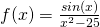?". According to the TAWFAE, this is actually a dumb question: TAWFAE says in order to know what a function is, we need to know what the domain is. So a question like doesn't actually contain enough information to be answerable.

What the question is really asking, though is: what's the most natural, or maybe what's the largest possible domain on which this rule makes sense?

As a middle-school student, I was very confused by questions like this one:

Find the domain of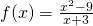.

My answer would usually go like this: that function is really the same as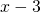, the domain of which is all of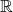. And I would always get that question wrong. Why?

The functions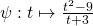and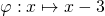do indeed have the same rule, in the sense that, whenevermakes sense, we have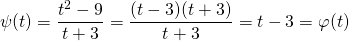But the natural domain of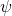is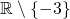and the natural domain for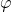is; so that means the domains are different -- hence by the TAWFAE, we know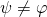. Nevertheless, the two functions are related.

Definition. If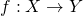and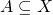, we define the restriction ofto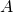as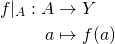That is,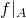has the same rule as, just we only care about inputs that come from.

Equipped with this notion, we can describe the relationship betweenand:Definition. If, and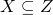, and there is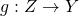with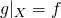, we callan extension of.

Here's an illustration: if we letdenote the function displayed in green,denote the function displayed in blue, and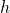the function displayed in red, then we have that:

•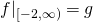•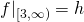•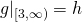•is an extension of, from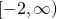to all of•is an extension of, from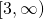to